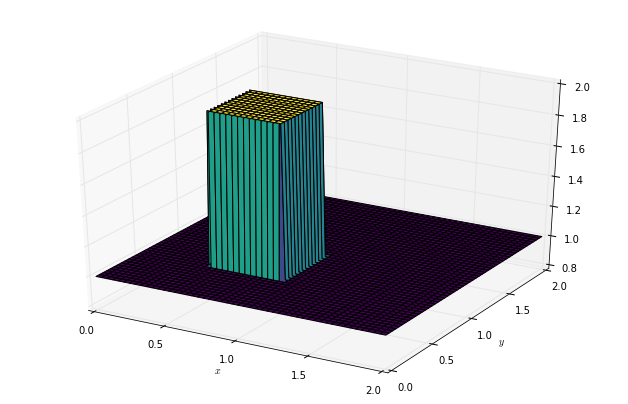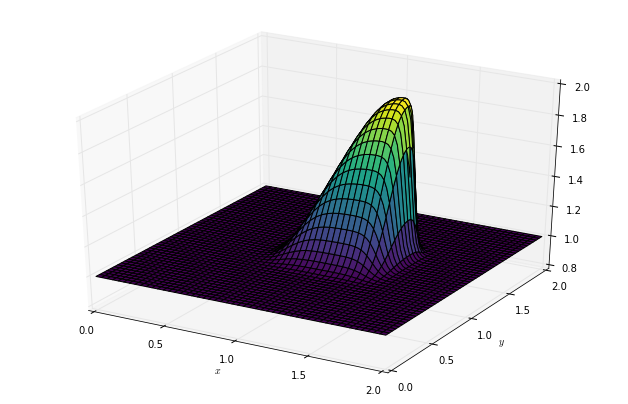Text provided under a Creative Commons Attribution license, CC-BY. All code is made available under the FSF-approved BSD-3 license. (c) Lorena A. Barba, Gilbert F. Forsyth 2017. Thanks to NSF for support via CAREER award #1149784.

# 12 steps to Navier–Stokes¶

You should have completed your own code for Step 5 before continuing to this lesson. As with Steps 1 to 4, we will build incrementally, so it's important to complete the previous step!

We continue ...

## Step 6: 2-D Convection¶

Now we solve 2D Convection, represented by the pair of coupled partial differential equations below:

$$\frac{\partial u}{\partial t} + u \frac{\partial u}{\partial x} + v \frac{\partial u}{\partial y} = 0$$$$\frac{\partial v}{\partial t} + u \frac{\partial v}{\partial x} + v \frac{\partial v}{\partial y} = 0$$

Discretizing these equations using the methods we've applied previously yields:

$$\frac{u_{i,j}^{n+1}-u_{i,j}^n}{\Delta t} + u_{i,j}^n \frac{u_{i,j}^n-u_{i-1,j}^n}{\Delta x} + v_{i,j}^n \frac{u_{i,j}^n-u_{i,j-1}^n}{\Delta y} = 0$$$$\frac{v_{i,j}^{n+1}-v_{i,j}^n}{\Delta t} + u_{i,j}^n \frac{v_{i,j}^n-v_{i-1,j}^n}{\Delta x} + v_{i,j}^n \frac{v_{i,j}^n-v_{i,j-1}^n}{\Delta y} = 0$$

Rearranging both equations, we solve for $u_{i,j}^{n+1}$ and $v_{i,j}^{n+1}$, respectively. Note that these equations are also coupled.

$$u_{i,j}^{n+1} = u_{i,j}^n - u_{i,j} \frac{\Delta t}{\Delta x} (u_{i,j}^n-u_{i-1,j}^n) - v_{i,j}^n \frac{\Delta t}{\Delta y} (u_{i,j}^n-u_{i,j-1}^n)$$$$v_{i,j}^{n+1} = v_{i,j}^n - u_{i,j} \frac{\Delta t}{\Delta x} (v_{i,j}^n-v_{i-1,j}^n) - v_{i,j}^n \frac{\Delta t}{\Delta y} (v_{i,j}^n-v_{i,j-1}^n)$$

### Initial Conditions¶

The initial conditions are the same that we used for 1D convection, applied in both the x and y directions.

$$u,\ v\ = \begin{cases}\begin{matrix} 2 & \text{for } x,y \in (0.5, 1)\times(0.5,1) \cr 1 & \text{everywhere else} \end{matrix}\end{cases}$$

### Boundary Conditions¶

The boundary conditions hold u and v equal to 1 along the boundaries of the grid .

$$u = 1,\ v = 1 \text{ for } \begin{cases} \begin{matrix}x=0,2\cr y=0,2 \end{matrix}\end{cases}$$
In :
from mpl_toolkits.mplot3d import Axes3D
from matplotlib import pyplot, cm
import numpy
%matplotlib inline

In :
###variable declarations
nx = 101
ny = 101
nt = 80
c = 1
dx = 2 / (nx - 1)
dy = 2 / (ny - 1)
sigma = .2
dt = sigma * dx

x = numpy.linspace(0, 2, nx)
y = numpy.linspace(0, 2, ny)

u = numpy.ones((ny, nx)) ##create a 1xn vector of 1's
v = numpy.ones((ny, nx))
un = numpy.ones((ny, nx))
vn = numpy.ones((ny, nx))

##set hat function I.C. : u(.5<=x<=1 && .5<=y<=1 ) is 2
u[int(.5 / dy):int(1 / dy + 1), int(.5 / dx):int(1 / dx + 1)] = 2
##set hat function I.C. : v(.5<=x<=1 && .5<=y<=1 ) is 2
v[int(.5 / dy):int(1 / dy + 1), int(.5 / dx):int(1 / dx + 1)] = 2

In :
fig = pyplot.figure(figsize=(11, 7), dpi=100)
ax = fig.gca(projection='3d')
X, Y = numpy.meshgrid(x, y)

ax.plot_surface(X, Y, u, cmap=cm.viridis, rstride=2, cstride=2)
ax.set_xlabel('$x$')
ax.set_ylabel('$y$');In :
for n in range(nt + 1): ##loop across number of time steps
un = u.copy()
vn = v.copy()
u[1:, 1:] = (un[1:, 1:] -
(un[1:, 1:] * c * dt / dx * (un[1:, 1:] - un[1:, :-1])) -
vn[1:, 1:] * c * dt / dy * (un[1:, 1:] - un[:-1, 1:]))
v[1:, 1:] = (vn[1:, 1:] -
(un[1:, 1:] * c * dt / dx * (vn[1:, 1:] - vn[1:, :-1])) -
vn[1:, 1:] * c * dt / dy * (vn[1:, 1:] - vn[:-1, 1:]))

u[0, :] = 1
u[-1, :] = 1
u[:, 0] = 1
u[:, -1] = 1

v[0, :] = 1
v[-1, :] = 1
v[:, 0] = 1
v[:, -1] = 1

In :
fig = pyplot.figure(figsize=(11, 7), dpi=100)
ax = fig.gca(projection='3d')
X, Y = numpy.meshgrid(x, y)

ax.plot_surface(X, Y, u, cmap=cm.viridis, rstride=2, cstride=2)
ax.set_xlabel('$x$')
ax.set_ylabel('$y$');In :
fig = pyplot.figure(figsize=(11, 7), dpi=100)
ax = fig.gca(projection='3d')
X, Y = numpy.meshgrid(x, y)
ax.plot_surface(X, Y, v, cmap=cm.viridis, rstride=2, cstride=2)
ax.set_xlabel('$x$')
ax.set_ylabel('$y$');The video lesson that walks you through the details for Steps 5 to 8 is Video Lesson 6 on You Tube:

In :
from IPython.display import YouTubeVideo

from IPython.core.display import HTML